# How to Combine Text and Formatted Values in Excel

In this article, we will learn How to Combine Text and Formatted Values in Excel.

Scenario:

Excel treats values to many formats like number, date, time, text, percentage or currency. These can be changed into each other. Excel converts numbers to text to use numbers as text in formulas like vlookup with numbers and text. The Excel TEXT function lets you convert the number to text. The TEXT function in Excel is used to convert numbers into text. The fun part is you can format that number to show it in the desired format. For example format a yy-mm-dd date into dd-mm-yy format. Add currency signs before a  number and many more.

TEXT formula in Excel

TEXT function is a string function which converts any value to a given format. The result may seem that it is a number but it's in text format.

 =TEXT(cell_ref, text_format)

cell_ref : value to convert using cell reference

Text_format : Format to convert

 Format Output_format d 7 ddd Thu dddd Thursday m 2 mmm Feb mmmm February mmmmm F yy 19 yyyy 2019 m/d/y 2/7/19 mm/dd/yy 02/07/19 mm/dd/yyyy 02/07/2019 ddd, mmm d Thu, Feb 7 mm/dd/yyyy h:mm AM/PM 02/07/2019 2:15 PM mm/dd/yyyy hh:mm:ss 07/02/2019 14:15:00

Example :

All of these might be confusing to understand. Let's understand how to use the function using an example. Here we have some examples to convert date values to text format or any other required format.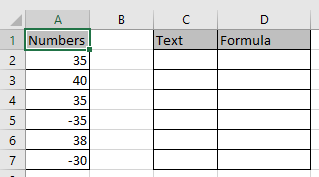Convert the value in A2 cell.

Use the formula in C2 cell

 =TEXT (A2, “0”)

“0”: returns the general text format.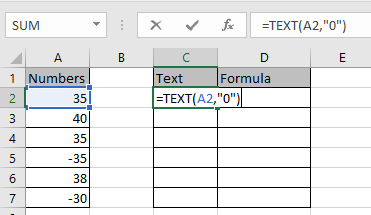Press Enter and copy the formula in remaining cells using Ctrl + D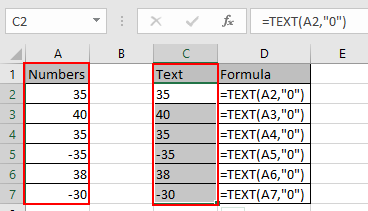As you can see we got numbers as text output because the significance number always varied.

Here we have some numbers to convert to text format or any other required format.

Use the formula:

 =TEXT(A2,"d")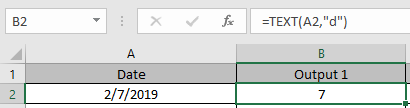As you can see the value in the output cell is in Text format.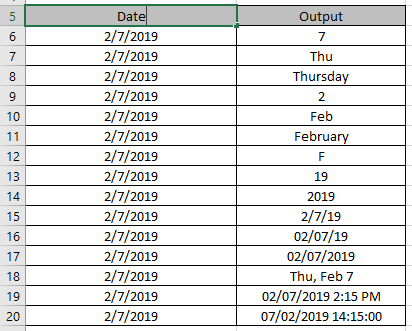You can use any Format_text and do your work in excel without any interruption

# Add 0 before a Number

Sometimes you are needed to add 0 before some fixed digit of numbers like phone number or pin number. Use this Text formula to do so…

 =TEXT( 1234, “00000”)

If you have N digits of number then in text format argument write n+1 0s.

# Add Currency Before a Number

Write this text formula to add currency.

 =TEXT( 1234, “\$0”)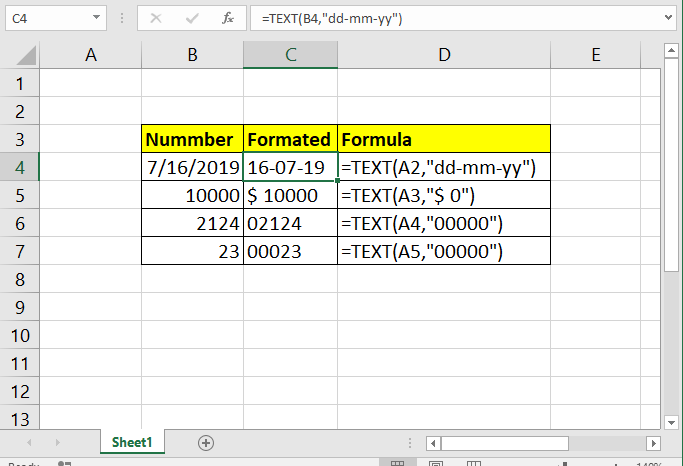As you can see we got numbers as text output because the significance number always varied.

VBA Code to convert currency in TEXT format

This code is divided into four individual functions. The main function is NUM_TO_IND_RUPEE_WORD. And other three functions GetHunderds(), GetTens() and GetDigits are helping function that help the main function to form the string.

Code

 Function NUM_TO_IND_RUPEE_WORD(ByVal MyNumber, Optional incRupees As Boolean = True)  Dim Crores, Lakhs, Rupees, Paise, Temp  Dim DecimalPlace As Long, Count As Long  Dim myLakhs, myCrores  ReDim Place(9) As String  Place(2) = " Thousand ": Place(3) = " Million "  Place(4) = " Billion ": Place(5) = " Trillion "    ' String representation of amount.  MyNumber = Trim(Str(MyNumber))    ' Position of decimal place 0 if none.  DecimalPlace = InStr(MyNumber, ".")    ' Convert Paise and set MyNumber to Rupees amount.  If DecimalPlace > 0 Then   Paise = GetTens(Left(Mid(MyNumber, DecimalPlace + 1) & "00", 2))   MyNumber = Trim(Left(MyNumber, DecimalPlace - 1))  End If    myCrores = MyNumber \ 10000000  myLakhs = (MyNumber - myCrores * 10000000) \ 100000  MyNumber = MyNumber - myCrores * 10000000 - myLakhs * 100000  Count = 1    Do While myCrores <> ""   Temp = GetHundreds(Right(myCrores, 3))   If Temp <> "" Then Crores = Temp & Place(Count) & Crores   If Len(myCrores) > 3 Then     myCrores = Left(myCrores, Len(myCrores) - 3)    Else     myCrores = ""   End If   Count = Count + 1  Loop  Count = 1    Do While myLakhs <> ""   Temp = GetHundreds(Right(myLakhs, 3))   If Temp <> "" Then Lakhs = Temp & Place(Count) & Lakhs   If Len(myLakhs) > 3 Then    myLakhs = Left(myLakhs, Len(myLakhs) - 3)   Else    myLakhs = ""   End If   Count = Count + 1  Loop  Count = 1    Do While MyNumber <> ""   Temp = GetHundreds(Right(MyNumber, 3))   If Temp <> "" Then Rupees = Temp & Place(Count) & Rupees   If Len(MyNumber) > 3 Then    MyNumber = Left(MyNumber, Len(MyNumber) - 3)   Else    MyNumber = ""   End If   Count = Count + 1  Loop    Select Case Crores   Case "": Crores = ""   Case "One": Crores = " One Crore "   Case Else: Crores = Crores & " Crores "  End Select  Select Case Lakhs   Case "": Lakhs = ""   Case "One": Lakhs = " One Lakh "   Case Else: Lakhs = Lakhs & " Lakhs "  End Select  Select Case Rupees   Case "": Rupees = "Zero "   Case "One": Rupees = "One "   Case Else:   Rupees = Rupees  End Select  Select Case Paise   Case "": Paise = " and Paise Zero Only "   Case "One": Paise = " and Paise One Only "   Case Else: Paise = " and Paise " & Paise & " Only "  End Select 'creating the string of words to translate number into words  NUM_TO_IND_RUPEE_WORD = IIf(incRupees, "Rupees ", "") & Crores & _ Lakhs & Rupees & Paise End Function ' Converts a number from 100-999 into text Function GetHundreds(ByVal MyNumber)  Dim Result As String  If Val(MyNumber) = 0 Then Exit Function  MyNumber = Right("000" & MyNumber, 3)  ' Convert the hundreds place.  If Mid(MyNumber, 1, 1) <> "0" Then  Result = GetDigit(Mid(MyNumber, 1, 1)) & " Hundred "  End If  ' Convert the tens and ones place.  If Mid(MyNumber, 2, 1) <> "0" Then   Result = Result & GetTens(Mid(MyNumber, 2))  Else   Result = Result & GetDigit(Mid(MyNumber, 3))  End If  GetHundreds = Result End Function ' Converts a number from 10 to 99 into text. Function GetTens(TensText)  Dim Result As String  Result = "" ' Null out the temporary function value.  If Val(Left(TensText, 1)) = 1 Then ' If value between 10-19...  Select Case Val(TensText)   Case 10: Result = "Ten"   Case 11: Result = "Eleven"   Case 12: Result = "Twelve"   Case 13: Result = "Thirteen"   Case 14: Result = "Fourteen"   Case 15: Result = "Fifteen"   Case 16: Result = "Sixteen"   Case 17: Result = "Seventeen"   Case 18: Result = "Eighteen"   Case 19: Result = "Nineteen"   Case Else  End Select  Else ' If value between 20-99...   Select Case Val(Left(TensText, 1))   Case 2: Result = "Twenty "   Case 3: Result = "Thirty "   Case 4: Result = "Forty "   Case 5: Result = "Fifty "   Case 6: Result = "Sixty "   Case 7: Result = "Seventy "   Case 8: Result = "Eighty "   Case 9: Result = "Ninety "  Case Else  End Select  Result = Result & GetDigit _  (Right(TensText, 1)) ' Retrieve ones place.  End If  GetTens = Result End Function ' Converts a number from 1 to 9 into text. Function GetDigit(Digit)  Select Case Val(Digit)   Case 1: GetDigit = "One"   Case 2: GetDigit = "Two"   Case 3: GetDigit = "Three"   Case 4: GetDigit = "Four"   Case 5: GetDigit = "Five"   Case 6: GetDigit = "Six"   Case 7: GetDigit = "Seven"   Case 8: GetDigit = "Eight"   Case 9: GetDigit = "Nine"   Case Else: GetDigit = ""  End Select End Function

The above code will get the result in correct format. Now make your own customized function.

#### Concatenating a text string and a formula-calculated value. To make the result returned by some formula more understandable for your users, you can concatenate it with a text string that explains what the value actually is.

For example, you can use the following formula to return the current date:

 =CONCATENATE("Today is ",TEXT(TODAY(), "dd-mmm-yy"))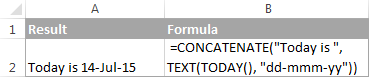Using CONCATENATE in Excel - things to remember

To ensure that your CONCATENATE formulas always deliver the correct results, remember the following simple rules:

Excel CONCATENATE function requires at least one "text" argument to work.

Here are all the observational notes using the formula in Excel
Notes :

1. Use & operator to combine text. & operator does the same work as CONCAT function
2. In new versions of Excel, CONCATENATE is replaced with the CONCAT function, which has exactly the same syntax. The CONCATENATE function is kept for backward compatibility, it is common practice to use CONCAT instead because Excel does not give any promises that CONCATENATE will be available in future versions of Excel.

Hope this article about How to Combine Text and Formatted Values in Excel is explanatory. Find more articles on calculating values and related Excel formulas here. If you liked our blogs, share them with your friends on Facebook. And also you can follow us on Twitter and Facebook. We would love to hear from you, do let us know how we can improve, complement or innovate our work and make it better for you. Write to us at info@exceltip.com.

Related Articles :

Excel REPLACE vs SUBSTITUTE function: The REPLACE and SUBSTITUTE functions are the most misunderstood functions. To find and replace a given text we use the SUBSTITUTE function. Where REPLACE is used to replace a number of characters in a string.

How to use the ISTEXT function in Excel : returns the TRUE logic value if the cell value is text using the ISTEXT function in Excel.

How to Highlight cells that contain specific text in Excel : Highlight cells based on the formula to find the specific text value within the cell in Excel.

Converts decimal Seconds into time format : As we know that time in excel is treated as numbers. Hours, Minutes, and Seconds are treated as decimal numbers. So when we have seconds as numbers, how do we convert into time format? This article got it covered.

Calculate Minutes Between Dates & Time in Excel : calculating the time difference is quite easy. Just need to subtract the start time from the end time. Learn more about this formula clicking the link

Replace text from end of a string starting from variable position : To replace text from the end of the string, we use the REPLACE function. The REPLACE function use the position of text in the string to replace.

Popular Articles :

How to use the VLOOKUP Function in Excel : This is one of the most used and popular functions of excel that is used to lookup value from different ranges and sheets.

How to use the IF Function in Excel : The IF statement in Excel checks the condition and returns a specific value if the condition is TRUE or returns another specific value if FALSE.

How to use the SUMIF Function in Excel : This is another dashboard essential function. This helps you sum up values on specific conditions.

How to use the COUNTIF Function in Excel : Count values with conditions using this amazing function. You don't need to filter your data to count specific values. Countif function is essential to prepare your dashboard.

1."Hi Sudhir,

Sounds like a one off thing that you will never need to replicate?

If so, to be honest, I suggest you do it manually.

With 50 to 60 sheets, I cannot imagine it will take more than an hour or so, and you could easily spend that long trying to write a macro or formulae that would do it for you.

Is that practical?

Alan."

2."i hv data in sheet1 from A1 to A3000 as mentioned below :
""Client Name"" ""Brand Name"" ""Bill No."" ""Bill Date""""Bill Amt.""

There are 50 to 60 sheets where columns are same but the data are different, now i hv to merge all sheets in one column, is there any formula? I hv tried ""consolidate"" formula in data menu but the problem is, it will only takes the total & other but i can't copy all text in one sheet? Could u pls help me?
Thanx & Regards, "

3."Hi Sudhir,

I doubt there will be a simple answer to this one.

Please can you give some samples so that we have a better idea of what you want to achieve.

Thanks,

Alan"

4.I hv 50 sheets in one file which has to be merged in one sheet, is there any formula to copy all sheets in one sheet?

5."Hi Sudhir,

Sounds like a one off thing that you will never need to replicate?

If so, to be honest, I suggest you do it manually.

With 50 to 60 sheets, I cannot imagine it will take more than an hour or so, and you could easily spend that long trying to write a macro or formulae that would do it for you.

Is that practical?

Alan."

6."i hv data in sheet1 from A1 to A3000 as mentioned below :
""Client Name"" ""Brand Name"" ""Bill No."" ""Bill Date""""Bill Amt.""

There are 50 to 60 sheets where columns are same but the data are different, now i hv to merge all sheets in one column, is there any formula? I hv tried ""consolidate"" formula in data menu but the problem is, it will only takes the total & other but i can't copy all text in one sheet? Could u pls help me?
Thanx & Regards, "

7."Hi Sudhir,

I doubt there will be a simple answer to this one.

Please can you give some samples so that we have a better idea of what you want to achieve.

Thanks,

Alan"

8.I hv 50 sheets in one file which has to be merged in one sheet, is there any formula to copy all sheets in one sheet?

Terms and Conditions of use

The applications/code on this site are distributed as is and without warranties or liability. In no event shall the owner of the copyrights, or the authors of the applications/code be liable for any loss of profit, any problems or any damage resulting from the use or evaluation of the applications/code.